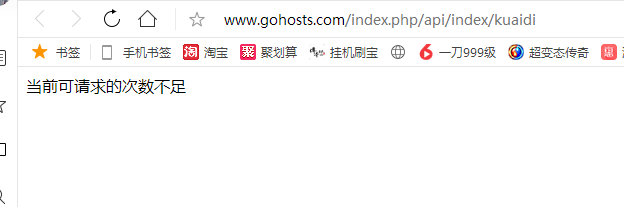# thinkphp框架resultful学习记录

1.快递查询实例

1.CURL函数库

Curl函数库的使用流程：

①使用curl_init初始化请求会话

②使用curl_setopt设置请求一些选项

③使用curl_exec执行，发送请求

④使用curl_close关闭请求会话

①在php.ini中开启php_curl扩展（必须开启）

②建议在php.ini中开启php_openssl扩展（本身不是curl必须的，是调用一些第三方接口需要的）

③如果以上操作重启apache后，curl还是不能使用，需要将php安装目录设置到环境变量。

if(!function_exists('curl_request')){
//发送curl请求
function curl_request($url,$type = false, $params = [],$https=false)
{
//调用curl_init() 初始化请求
$ch = curl_init($url);
//调用curl_setopt()设置请求选项
if($type){ //true 发送post请求 false 默认发送get请求 //post请求 设置请求方式 curl_setopt($ch, CURLOPT_POST, true);
//设置请求参数
curl_setopt($ch, CURLOPT_POSTFIELDS,$params);
}
//如果是https请求 需要禁止从服务器端验证本地的证书
if($https){ curl_setopt($ch, CURLOPT_SSL_VERIFYPEER, false);
}
//调用curl_exec() 发送请求 获取结果
curl_setopt($ch, CURLOPT_RETURNTRANSFER, true);$res = curl_exec($ch); // if(!$res){
//            //错误信息
//            $error = curl_error($ch);
//            //错误码
//            $errno = curl_errno($ch);
//        }
//调用curl_close() 关闭请求
curl_close($ch); return$res;
}
}

api模块index控制器kuaidi方法

public function kuaidi()
{
//请求地址
$url="http://v.juhe.cn/exp/index?com=zto&no=73115984252335&key=ac2dde994cc76d4f15738f7f97af7ca4"; //请求方式 //请求参数get //请求参数已经拼接到url中 //发送请求$res=curl_request($url); if(!$res){
echo '请求错误';die;
}
//解析数据
$arr=json_decode($res,true);
if($arr['resultcode']!= 200){ echo$arr['reason'];die;
echo '查询失败';die;
}
//获取物流进度数据
$list=$arr['result']['list'];
echo '时间----------进度<br>';
foreach ($list as$v){
echo $v['datetime'],'--------------',$v['remark'],'<br>';
}
die;
}posted @ 2020-10-15 22:28  小高家的小胖子  阅读(144)  评论(0编辑  收藏  举报